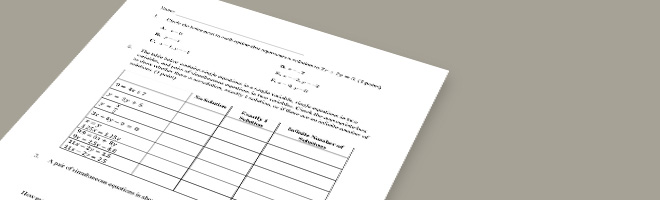• 03/04/14   |   Adjusted: 10/09/19   |   2 files# Simultaneous Linear Equations Mini-Assessment

Author: Student Achievement Partners

• Description
• Files

This mini-assessment is designed to illustrate the standard 8.EE.C.8, particularly 8.EE.C.8.a and 8.EE.C.8.c, which sets an expectation for understanding and applying pairs of simultaneous linear equations (systems of equations). It consists of a variety of carefully chosen problem types, including fill-in-the-blank, multiple choice, short answer, and constructed response, in order to address the breadth of understanding and applying pairs of simultaneous linear equations. This mini-assessment is designed for teachers to use either in the classroom, for self-learning, or in professional development settings to:

• Gain knowledge about assessing conceptual understanding and application of pairs of simultaneous linear equations;
• Use in professional development as an illustration of CCSS-aligned assessment problems; and
• Identify strengths and weaknesses in students' understandings of the content in 8.EE.C.8.a and 8.EE.C.8.c – whether before, during, or after teaching aspects of this material.

• Making the Shifts

How does this mini-assessment exemplify the instructional Shifts required by CCSSM?

 Focus Belongs to the major work of eighth grade Coherence Builds on work around understanding and using ratios and proportional relationships (7.RP) and using equations for problem solving (7.EE.A); sets the stage for more abstract work in algebra, functions, and modeling Rigor Conceptual Understanding: primary in this mini-assessment Procedural Skill and Fluency: not targeted in this mini-assessment Application: primary in this mini-assessment
• Noteworthy features of this resource
• Mathematically:
• Focuses on linear equations and simultaneous linear equations
• Uses application problems derived from constraint-based situations that require equations
• Challenges students to choose whether a linear equation or system of linear equations correctly models a mathematical situation
• As a mini-assessment:
• Assesses important concepts directly, like knowing that a solution to a single equation in two variables is a pair of numbers that makes the equation true
• Uses a mix of short conceptual problems with problems that require longer chains of reasoning
• Allows the teacher to evaluate students on their understanding of 8.EE.C.8a and 8.EE.C.8c in order to prepare to teach 8.EE.C.8a and 8.EE.C.8c or to check student understanding after teaching 8.EE.C.8a and 8.EE.C.8c
• Shows equations in various ways (e.g., $1.25x=1.25y$, $9.9=6x+8y$, $9x=2.5y-8.8$, etc.) to ensure students have conceptual understanding in addition to procedural knowledge